Python语言学习之Python深入06 Python的内存管理#### 语言的内存管理

语言的内存管理是语言设计的一个重要方面。它是决定语言性能的重要因素。无论是C语言的手工管理，还是Java的垃圾回收，都成为语言最重要的特征。这里以Python语言为例子，说明一门动态类型的、面向对象的语言的内存管理方式。

#### 对象的内存使用

赋值语句是语言最常见的功能了。但即使是最简单的赋值语句，也可以很有内涵。Python的赋值语句就很值得研究

`a=1`

整数1为一个对象。而a是一个引用。利用赋值语句，引用a指向对象1。Python是动态类型的语言(参考动态类型)，对象与引用分离。Python像使用“筷子”那样，通过引用来接触和翻动真正的食物——对象#### 引用和对象

为了探索对象在内存的存储，我们可以求助于Python的内置函数`id()`。它用于返回对象的身份(identity)。其实，这里所谓的身份，就是该对象的`内存地址`

`a = 1`
`print(id(a))`
`print(hex(id(a)))`

在我的计算机上，它们返回的是:

`11246696`
`'0xab9c68'`

分别为内存地址的十进制和十六进制表示。

在Python中，整数和短小的字符，Python都会缓存这些对象，以便重复使用。当我们创建多个等于1的引用时，实际上是让所有这些引用指向同一个对象。

`a = 1`
`b = 1`
`print(id(a))`
`print(id(b))`

`11246696`

`11246696`

可见a和b实际上是指向同一个对象的两个引用。

为了检验两个引用指向同一个对象，我们可以用is关键字。is用于判断两个引用所指的对象是否相同。

`True`
`a = 1`
`b = 1`
`print(a is b)`
`True`
`a = ""good""`
`b = ""good""`
`print(a is b)`
`False`
`a = ""very good morning""`
`b = ""very good morning""`
`print(a is b)`
`False`
`a = []`
`b = []`
`print(a is b`

上面的注释为相应的运行结果。可以看到，由于Python缓存了整数和短字符串，因此每个对象只存有一份。比如，所有整数1的引用都指向同一对象。即使使用赋值语句，也只是创造了新的引用，而不是对象本身。长的字符串和其它对象可以有多个相同的对象，可以使用赋值语句创建出新的对象。

在Python中，每个对象都有存有指向该对象的引用总数，即引用计数(`reference count`)。

我们可以使用sys包中的`getrefcount()`，来查看某个对象的引用计数。需要注意的是，当使用某个引用作为参数，传递给`getrefcount()`时，参数实际上创建了一个临时的引用。因此，`getrefcount()`所得到的结果，会比期望的多1。

`from sys import getrefcount`
`a = [1, 2, 3]`
`print(getrefcount(a))`
`b = a`
`print(getrefcount(b))`

由于上述原因，两个getrefcount将返回2和3，而不是期望的1和2。

#### 对象引用对象

Python的一个容器对象(container)，比如表、词典等，可以包含多个对象。实际上，容器对象中包含的并不是元素对象本身，是指向各个元素对象的引用。

`class from_obj(object):`
`def __init__(self, to_obj):`
`self.to_obj = to_obj`
`b = [1,2,3]`
`a = from_obj(b)`
`print(id(a.to_obj))`
`print(id(b))`

对象引用对象，是Python最基本的构成方式。即使是a = 1这一赋值方式，实际上是让词典的一个键值""a""的元素引用整数对象1。该词典对象用于记录所有的全局引用。该词典引用了整数对象1。我们可以通过内置函数globals()来查看该词典。

`from sys import getrefcount`
`a = [1, 2, 3]`
`print(getrefcount(a))`
`b = [a, a]`
`print(getrefcount(a))`

`x = [1, 2, 3]`
`y = [x, dict(key1=x)]`
`z = [y, (x, y)]`
`import objgraph`
`objgraph.show_refs([z], filename='ref_topo.png')`

objgraph是Python的一个第三方包。安装之前需要安装xdot。

sudo apt-get install xdot
sudo pip install objgraph

`a = []`
`b = [a]`
`a.append(b)`

`a = []`
`a.append(a)`
`print(getrefcount(a))`

#### 引用减少

`from sys import getrefcount`
`a = [1, 2, 3]`
`b = a`
`print(getrefcount(b))`
`del a`
`print(getrefcount(b))`

del也可以用于删除容器元素中的元素，比如:

`a = [1,2,3]`
`del a`
`print(a)`

`from sys import getrefcount`
`a = [1, 2, 3]`
`b = a`
`print(getrefcount(b))`
`a = 1`
`print(getrefcount(b))`

# 垃圾回收

吃太多，总会变胖，Python也是这样。当Python中的对象越来越多，它们将占据越来越大的内存。不过你不用太担心Python的体形，它会乖巧的在适当的时候“减肥”，启动`垃圾回收`(garbage collection)，将没用的对象清除。在许多语言中都有垃圾回收机制，比如Java和Ruby。尽管最终目的都是塑造苗条的提醒，但不同语言的减肥方案有很大的差异 (这一点可以对比本文和Java内存管理与垃圾回收

从基本原理上，当Python的某个对象的引用计数降为0时，说明没有任何引用指向该对象，该对象就成为要被回收的垃圾了。比如某个新建对象，它被分配给某个引用，对象的引用计数变为1。如果引用被删除，对象的引用计数为0，那么该对象就可以被垃圾回收。比如下面的表:

`a = [1, 2, 3]`
`del a`

del a后，已经没有任何引用指向之前建立的[1, 2, 3]这个表。用户不可能通过任何方式接触或者动用这个对象。这个对象如果继续待在内存里，就成了不健康的脂肪。当垃圾回收启动时，Python扫描到这个引用计数为0的对象，就将它所占据的内存清空。

然而，减肥是个昂贵而费力的事情。垃圾回收时，Python不能进行其它的任务。频繁的垃圾回收将大大降低Python的工作效率。如果内存中的对象不多，就没有必要总启动垃圾回收。所以，Python只会在特定条件下，`自动启动`垃圾回收。当Python运行时，会记录其中分配对象(object allocation)和取消分配对象(object deallocation)的次数。当两者的差值高于某个`阈值`时，垃圾回收才会启动。

`import gc`
`print(gc.get_threshold())`

#### 分代回收

Python同时采用了`分代`(generation)回收的策略。这一策略的基本假设是，存活时间越久的对象，越不可能在后面的程序中变成垃圾。我们的程序往往会产生大量的对象，许多对象很快产生和消失，但也有一些对象长期被使用。出于信任和效率，对于这样一些“长寿”对象，我们相信它们的用处，所以减少在垃圾回收中扫描它们的频率。

Python将所有的对象分为0，1，2三代。所有的新建对象都是0代对象。当某一代对象经历过垃圾回收，依然存活，那么它就被归入下一代对象。垃圾回收启动时，一定会扫描所有的0代对象。如果0代经过一定次数垃圾回收，那么就启动对0代和1代的扫描清理。当1代也经历了一定·次数·的垃圾回收后，那么会启动对0，1，2，即对所有对象进行扫描。

这两个次数即上面get_threshold()返回的(700, 10, 10)返回的两个10。也就是说，每10次0代垃圾回收，会配合1次1代的垃圾回收；而每10次1代的垃圾回收，才会有1次的2代垃圾回收。

`import gc`
`gc.set_threshold(700, 10, 5)`

#### 孤立的引用环

`a = []`
`b = [a]`
`a.append(b)`
`del a`
`del b`

上面我们先创建了两个表对象，并引用对方，构成一个引用环。删除了a，b引用之后，这两个对象不可能再从程序中调用，就没有什么用处了。但是由于引用环的存在，这两个对象的引用计数都没有降到0，不会被垃圾回收。

#### 孤立的引用环

为了回收这样的引用环，Python复制每个对象的引用计数，可以记为`gc_ref`。假设，每个对象i，该计数为`gc_ref_i`。Python会遍历所有的对象i。对于每个对象i引用的对象j，将相应的`gc_ref_j`减1。

#### 总结

Python作为一种动态类型的语言，其对象和引用分离。这与曾经的面向过程语言有很大的区别。为了有效的释放内存，Python内置了垃圾回收的支持。Python采取了一种相对简单的垃圾回收机制，即引用计数，并因此需要解决孤立引用环的问题。Python与其它语言既有共通性，又有特别的地方。对该内存管理机制的理解，是提高Python性能的重要一步。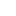喜欢 | 0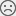不喜欢 | 0

• 10
文章
• 1016
人气
• 100%
受欢迎度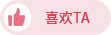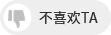•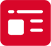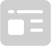索取资料
•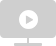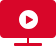答疑解惑
•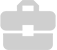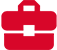技术交流
•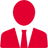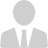职业测评
•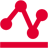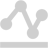面试技巧
•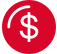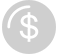高薪秘笈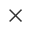Java全栈开发
WEB前端+H5沪公网安备 31011502005948号    ICP许可  沪B2-20190160